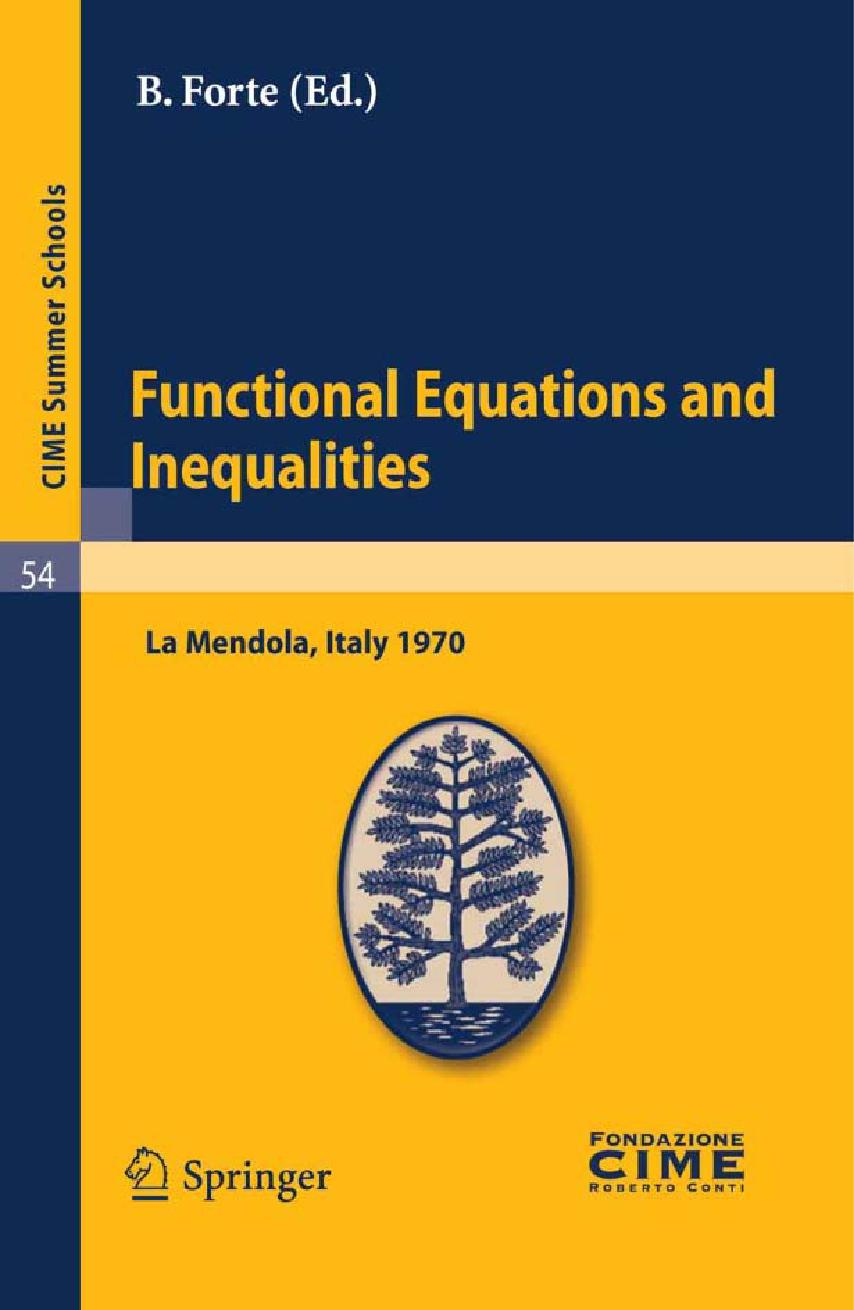### Book Toankho

Sách hay - Sách đẹp

# Functional Equations and InequalitiesJ. Aczél: Some applications of functional equations and inequalities to information measures.- J.A. Baker: Functional equations in vector space, part II.- I Fenyo: Sur les équations distributionnelles.- B. Forte: Applications of functional equations and inequalities to information theory.- S. Golab: Sur l’équation fonctionnelle des brigade.- E. Hille: Mean-values and functional equations.- J. Kampé de Feriet: Applications of functional equations and inequalities to information theory. Measure of information by a set of observers: a functional equation.- M. Kuczma: Convex functions.- S. Kurepa: Functional equations on vector spaces.- E. Lukacs: Inequalities and functional equations in probability theory.- M.A. McKiernan: Difference and mean-value type functional equations.- T.S. Motzkin: Solutions of differential and functional inequalities.- C.T. Ng: Uniqueness theorems in the theory of functional equations and related homotopy.- A.M. Ostrowski: Integral inequalities.- H. Schwerdtfeger: Remark on an inequality for monotonic functions.